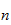StatLect

# Sample size

In statistical inference, the set of observed data that is used to draw inferences is called sample, and the number of observations in the sample is called sample size.## Definition

A more accurate definition follows.

Definition Suppose a sampleis made ofrealizationsofrandom variables - or random vectors. Then we say that the sample has sizeor that the sample size is.

## Not to be confused with the size of a test

Note that in statistical inference there is another concept, the size of a statistical test, that must not be confused with the sample size.

The size of a statistical test is the (maximum) probability of incorrectly rejecting the null hypothesis when the null hypothesis is true.

## How the sample size affects statistical estimation

As a general rule, the smallest the sample size is, the less reliable the statistical inferences drawn from the sample are.

For example, in mean estimation, the variance of the estimate is inversely proportional to the sample size. In other words, the smallest the sample size is, the less precise the estimate is, and the larger the confidence interval attached to the estimate is (see the lecture on interval estimation of the mean for details).

## Small vs large samples

When the sample sizetends to infinity, the properties of the statistical inferences that are drawn by using the sample can be studied using asymptotic results such as the Law of Large Numbers and the Central Limit Theorem.

A sample is called a large sample when the sample size is so large that the asymptotic properties (i.e., those that are valid forthat tends to infinity) are deemed a very good approximation of the actual properties enjoyed by the sample.

On the contrary, when the sample size is not sufficient to justify such an approximation, the sample is called a small sample.

## How large is a large sample?

When isso large that we can rely on asymptotic properties?

Unfortunately, there is no general answer to this question and how good the asymptotic approximation is should be judged on the basis of Monte Carlo simulations, as is done in most of the academic papers that deal with large sample approximations.

However, if you consult the internet or the applied statistics literature, you will find that several rules of thumb are proposed, for example, that the sample size should be greater than 30 or 50 for large sample results to approximately hold. These rules of thumb are usually derived under very special assumptions and have no general validity.

## More details

You can go to the lecture entitled Statistical inference to read more details about samples and sample size.

Previous entry: Sample point

Next entry: Sample space